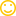## Sunday, September 28, 2014

We all know that Imaginary Mathematical Proved are called A Fallacy .
Previously we share

## How to Prove 1=2 ? [Solved]

Now, we share another Mathematical Fallacy i.e.  2 + 2 = 5

We know that : -20 = -20
Which is the same as: 16-36 = 25-45
Which can also be expressed as: (2+2) 2 (9 X (2+2) = 52) 9 X 5
Add 81/4 to both sides: (2+2) 2 (9 X (2+2) + 81/4 = 52) 9 X 5 + 81/4
Rearrange the terms: ({2+2}) 9/2) 2 = (5-9/2) 2
Ergo: 2+2 - 9/2 = 5
Hence: 2 + 2 = 5

See... simple, isn't it?Note :
See if you can figure out in which step the fallacy lies. When you think you've figured it out,comment it we will tell you whether you are correct or not, and will give an additional explanation of why that step is or isn't valid.
See how many tries it takes you to correctly identify the fallacious step!